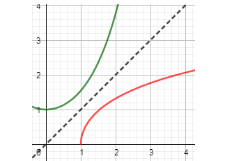QuestionAnswers

# The two curves shown in the below figure with $\cosh \left( x \right)$ as green colored and ${{\cosh }^{-1}}\left( x \right)$ as red colored are correctly labeled.Is the above statement true or false?Verified
130.5k+ views
Hint: We know that $\cosh \left( x \right)\And {{\cosh }^{-1}}\left( x \right)$ both are hyperbolic functions with $\cosh \left( x \right)=\dfrac{{{e}^{x}}+{{e}^{-x}}}{2}$ and ${{\cosh }^{-1}}\left( x \right)=\ln \left( x+\sqrt{{{x}^{2}}-1} \right)$ . Now, find the minimum value of $\cosh \left( x \right)\And {{\cosh }^{-1}}\left( x \right)$ from the equation that we have just described by putting $x=0$ in these equations and then compare the minimum values from the graph shown in the above problem. If the minimum values of $\cosh \left( x \right)\And {{\cosh }^{-1}}\left( x \right)$ from the equations are matched with the graph given above, then the curves are correctly labeled.

The below graph shown in the above question has two curves marked with red and green colour.It is given that green color curve corresponds to $\cosh \left( x \right)$ and red color corresponds to ${{\cosh }^{-1}}\left( x \right)$ and we have to show whether the labeling of the curves are correct or not.
We know that $\cosh \left( x \right)\And {{\cosh }^{-1}}\left( x \right)$ are hyperbolic functions. In the below, we are showing the hyperbolic functions in x corresponding to $\cosh \left( x \right)\And {{\cosh }^{-1}}\left( x \right)$ .
The function of $\cosh \left( x \right)$ is equal to:
$\cosh \left( x \right)=\dfrac{{{e}^{x}}+{{e}^{-x}}}{2}$ …………. Eq. (1)
The function of ${{\cosh }^{-1}}\left( x \right)$ is equal to:
${{\cosh }^{-1}}\left( x \right)=\ln \left( x+\sqrt{{{x}^{2}}-1} \right)$ ……….. Eq. (2)
Now, to check whether the curves labeled in the above question are correct or not by finding the minimum values of $\cosh \left( x \right)\And {{\cosh }^{-1}}\left( x \right)$ .
Substituting $x=0$ in eq. (1) we get,
\begin{align} & \cosh \left( 0 \right)=\dfrac{{{e}^{\left( 0 \right)}}+{{e}^{\left( 0 \right)}}}{2} \\ & \Rightarrow \cosh \left( 0 \right)=\dfrac{1+1}{2} \\ & \Rightarrow \cosh \left( 0 \right)=\dfrac{2}{2}=1 \\ \end{align}
Now, at $x=0$ we have got the value of $\cosh \left( x \right)$ as 1 which is the same as given in the above problem.
Hence, the green curve corresponding to $\cosh \left( x \right)$ is correctly labeled.
If we assume that what is given in the question is right i.e. red curve corresponds to ${{\cosh }^{-1}}\left( x \right)$ then the minimum value of this function occurs at $x=1$ and is 0 so let us substitute $x=1$ in eq. (2) to see whether the value of ${{\cosh }^{-1}}\left( x \right)$ is coming 0 or not.
\begin{align} & {{\cosh }^{-1}}\left( x \right)=\ln \left( x+\sqrt{{{x}^{2}}-1} \right) \\ & \Rightarrow {{\cosh }^{-1}}\left( 1 \right)=\ln \left( 1+\sqrt{{{1}^{2}}-1} \right) \\ \end{align}
\begin{align} & \Rightarrow {{\cosh }^{-1}}\left( 1 \right)=\ln \left( 1+\sqrt{0} \right) \\ & \Rightarrow {{\cosh }^{-1}}\left( 1 \right)=\ln \left( 1 \right) \\ \end{align}
And we know that, the value of $\ln \left( 1 \right)=0$ so the value of the above equation becomes:
${{\cosh }^{-1}}\left( 1 \right)=0$
Hence, we have got the same minimum value of ${{\cosh }^{-1}}\left( x \right)$ which is given in the above problem. Hence, the red curve which is labeled as ${{\cosh }^{-1}}\left( x \right)$ is correct.
From the above, we can say that the above statement is true.

Note: Don’t confuse the functions $\cosh \left( x \right)\And {{\cosh }^{-1}}\left( x \right)$ given in the above problem with $\cos x\And {{\cos }^{-1}}\left( x \right)$ . These two functions are completely different from each other. The functions $\cosh \left( x \right)\And {{\cosh }^{-1}}\left( x \right)$ are hyperbolic functions whereas $\cos x\And {{\cos }^{-1}}\left( x \right)$ are trigonometric functions.
Usually, students ignore the “h” written with cosine and think it might be a typo but it is not so make sure you don’t repeat such mistakes.